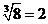# Exponents & Roots

### Exponents & Roots

1. Exponents

Exponents are used to represent the repeated multiplication of a number by itself. Some rules of exponents are given below: –For example, 23 = 2 × 2 × 2  = 8
In the expression, 23, 2 is called the base, 3 is called the exponent, and we read the expression as “2 to the third power.”

When the exponent is 2, we call the process squaring.
For example,
52 = 25, is read as “5 squared is 25”.
62 = 36, is read as “6 squared is 36”.

When negative numbers are raised to powers, the result may be positive or negative. A negative number raised to an even power is always positive, and a negative number raised to an odd power is always negative.

For example,
(−3)4 = −3 × −3 × −3 × −3 = 81
(−3)3= −3 × −3 × −3 = −27

Take note of the parenthesis: (−3)2 = 9, but −32 = −9

2. Square Roots

A square root of a non-negative number n is a number r such that r2 = n.
For example, 5 is a square root of 25 because 52 = 25.
Another square root of 25 is −5 because (−5)2 is also equals to 25.

### Rules for Square Roots

Here are some important rules regarding operations with square roots, where x > 0 and y > 0For example,
8 has one cube root.The cube root of 8 is 2 because 23 = 8.
−8 has one cube root.The cube root of −8 is −2 because (−2)3= −8

8 has two fourth roots.because 24 = 16 and (−2)4 = 16

nc